## Estimating quantities from several hundred to several thousand

How much influence do anchoring and financial incentives have on estimation accuracy?

Anchoring is a cognitive bias which occurs when a decision is influenced by irrelevant information. For instance, a study by John Horton asked 196 subjects to estimate the number of dots in a displayed image, but before providing their estimate subjects had to specify whether they thought the number of dots was higher/lower than a number also displayed on-screen (this was randomly generated for each subject).

How many dots do you estimate appear in the plot below?Estimates are often round numbers, and 46% of dot estimates had the form of a round number. The plot below shows the anchor value seen by each subject and their corresponding estimate of the number of dots (the image always contained five hundred dots, like the one above), with round number estimates in same color rows (e.g., 250, 300, 500, 600; code+data):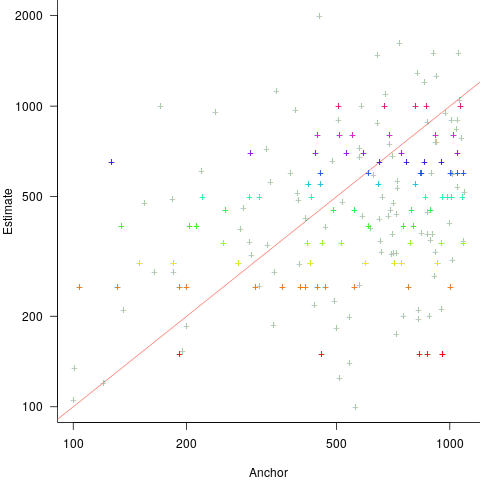How much influence does the anchor value have on the estimated number of dots?

One way of measuring the anchor’s influence is to model the estimate based on the anchor value. The fitted regression equation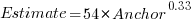explains 11% of the variance in the data. If the higher/lower choice is included the model, 44% of the variance is explained; higher equation is: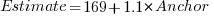and lower equation is: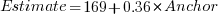(a multiplicative model has a similar goodness of fit), i.e., the anchor has three-times the impact when it is thought to be an underestimate.

How much would estimation accuracy improve if subjects’ were given the option of being rewarded for more accurate answers, and no anchor is present?

A second experiment offered subjects the choice of either an unconditional payment of \$2.50 or a payment of \$5.00 if their answer was in the top 50% of estimates made (labelled as the risk condition).

The 196 subjects saw up to seven images (65 only saw one), with the number of dots varying from 310 to 8,200. The plot below shows actual number of dots against estimated dots, for all subjects; blue/green line shows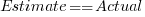, and red line shows the fitted regression model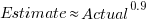(code+data):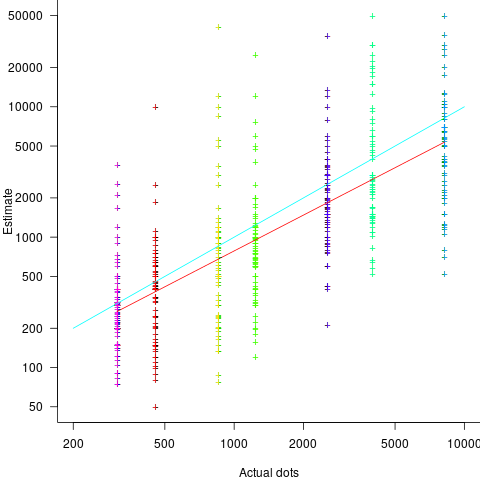The variance in the estimated number of dots is very high and increases with increasing actual dot count, however, this behavior is consistent with the increasing variance seen for images containing under 100 dots.

Estimates were not more accurate in those cases where subjects chose the risk payment option. This is not surprising, performance improvements require feedback, and subjects were not given any feedback on the accuracy of their estimates.

Of the 86 subjects estimating dots in three or more images, 44% always estimated low and 16% always high. Subjects always estimating low/high also occurs in software task estimates.

Estimation patterns previously discussed on this blog have involved estimated values below 100. This post has investigated patterns in estimates ranging from several hundred to several thousand. Patterns seen include extensive use of round numbers and increasing estimate variance with increasing actual value; all seen in previous posts.

## Rounding and heaping in non-software estimates

Round numbers are often preferred in software task estimation times, e.g., 1, 5, 7 (hours in one working day), and 14. This human preference for round numbers is not specific to software, or to estimating. Round numbers can act as goals, as clustering points, may be used more often as uncertainty increases, or be the result of satisficing, etc.

Rounding can occur in response to any question involving a numeric value, e.g., a government census or survey asking citizens about their financial situation or health. Rounding introduces error in the analysis of data. The Whipple index, described in 1919, was the first attempt to quantify the amount of error; calculated as: “per cent which the number reported as multiples of 5 forms of one-fifth of the total number between ages 23 to 62 years inclusive.” for errors of reported age. Other metrics for this error have been proposed, and packages to calculate them are available.

At some point (the evidence suggests a 1940 paper) a published paper introduced the term heaping effect. These days, heaping is more often used to name the process, compared to rounding, e.g., heaping of values; ‘heaping’ papers do use the term rounding, but I have not seen ’rounding’ papers use heaping.

The choice of rounding values depends on the unit of measurement. For instance, reported travel arrival/departure times are rounded to intervals of 5, 14, 30 and 60 minutes; based on reported/actual travel times it is possible to estimate the probability that particular rounding intervals have been used.

The Whipple index fails when all the values are large (e.g., multiple thousands), or take a small range of values (e.g., between one and twenty).

One technique for handling rounding of large values is to define roundedness in terms of the fraction of value digits that are trailing zeroes. The plot below shows the number of households having a given estimated balance on their first mortgage in the 2013 Survey of Consumer Finances (in red), and the distribution of actual balances reported by the New York Federal Reserve (in blue/green; data extracted from plot in a paper and scaled to equalize total mortgage values; code+data):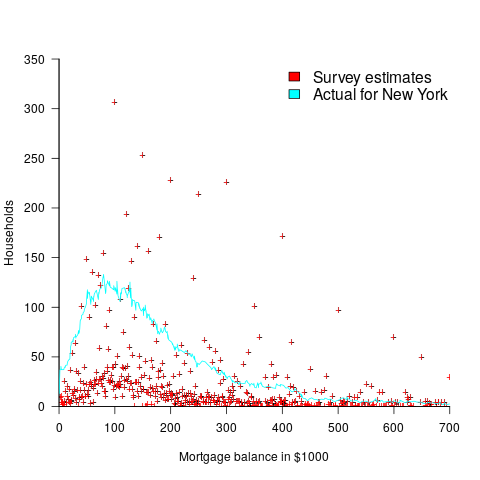The relatively high number of distinct round numbers swamps any underlying distribution of actual values. While some values having some degree of roundness occur more often than non-round values, they still appear less often than expected by the known distribution. It is possible that homeowners have mortgages at round values because they of banking limits, or reasons other than rounding when answering the survey.

The plot below shows the number of people reporting having a given number of friends, plus number of cigarettes smoked per day, from the 2015 survey of Objective and Subjective Quality of Life in Poland (code+data):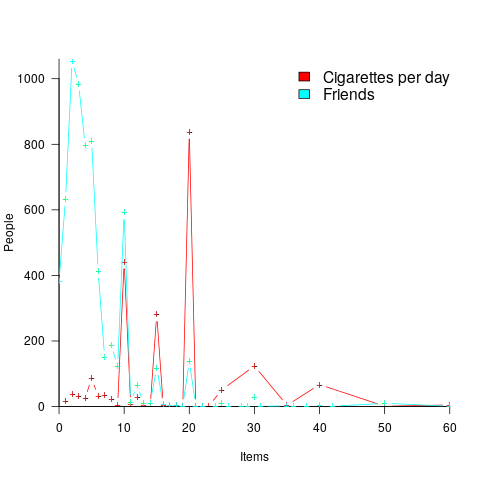The narrow range of a person’s number of friends prevents the Whipple index from effectively detecting rounding/heaping.

The dominance of round numbers in the cigarettes smoked per day may be caused by the number of cigarettes contained in a packet, i.e., people may be accurately reporting that they smoke the contents of a packet, rather than estimating a rounded number.

Simple techniques are available for correcting the mean/variance when values are always rounded to specified boundaries. When the probability of rounding is not 100%, the calculation is more complicated.

Rounded/Heaped data contains multiple distributions, i.e., the non-rounded values and the rounded values; various mixture models have been proposed to fit such data. Alternatively, the data can be ‘deheaped’, and various deheaping techniques have been proposed.

Given the prevalence of significant amounts of rounding/heaping, it’s surprising how few people know about it.

## The Approximate Number System and software estimating

The ability to perform simple numeric operations can improve the fitness of a creature (e.g., being able to select which branch contains the most fruit), increasing the likelihood of it having offspring. Studies have found that a wide variety of creatures have a brain subsystem known as the Approximate Number System (ANS).

A study by Mechner rewarded rats with food, if they pressed a lever `N` times (with `N` taking one of the values 4, 8, 12 or 16), followed by pressing a second lever. The plot below shows the number of lever presses made before pressing the second lever, for a given required `N`; it suggests that the subject rat is making use of an approximate number system (code+data):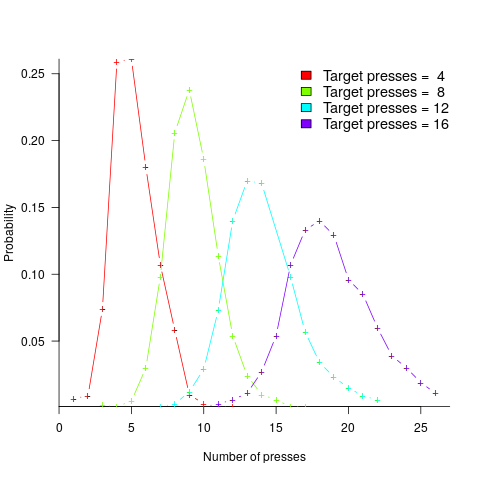Humans have a second system for representing numbers, which is capable of exact representation, it is language. The Number Sense by Stanislas Dehaene was on my list of Christmas books for 2011.

One method used to study the interface between the two language systems, available to humans, involves subjects estimating the number of dots in a briefly presented image. While reading about one such study, I noticed that some of the plots showed patterns similar to the patterns seen in plots of software estimate/actual data. I emailed the lead author, Véronique Izard, who kindly sent me a copy of the experimental data.

The patterns I was hoping to see are those invariably seen in software effort estimation data, e.g., a power law relationship between actual/estimate, consistent over/under estimation by individuals, and frequent use of round numbers.

Psychologists reading this post may be under the impression that estimating the time taken to implement some functionality, in software, is a relatively accurate process. In practice, for short tasks (i.e., under a day or two) the time needed to form a more accurate estimate makes a good-enough estimate a cost-effective option.

This Izard and Dehaene study involved two experiments. In the first experiment, an image containing between 1 and 100 dots was flashed on the screen for 100ms, and subjects then had to type the estimated number of dots. Each of the six subjects participated in five sessions of 600 trials, with each session lasting about one hour; every number of dots between 1 and 100 was seen 30 times by each subject (for one subject the data contains 1,783 responses, other subjects gave 3,000 responses). Subjects were free to type any value as their estimate.

These kinds of studies have consistently found that subject accuracy is very poor (hardly surprising, given that subjects are not provided with any feedback to help calibrate their estimates). But since researchers are interested in patterns that might be present in the errors, very low accuracy is not an issue.

The plot below shows stimulus (number of dots shown) against subject response, with green line showing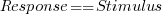, and red line a fitted regression model having the form(which explains just over 70% of the variance; code+data):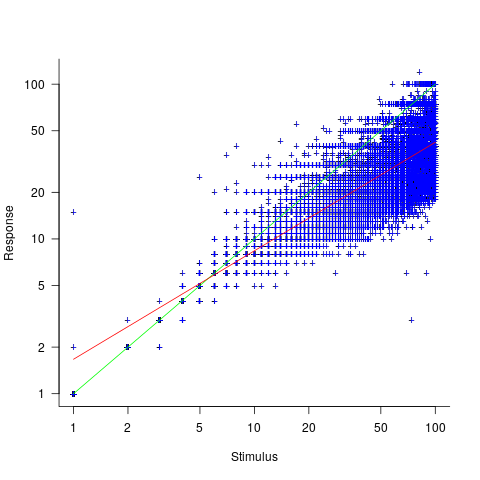Just like software estimates, there is a good fit to a power law, and the only difference in accuracy performance is that software estimates tend not to be so skewed towards underestimating (i.e., there are a lot more low accuracy overestimates).

Adding subjectID to the model gives:, withvarying between 0.65 and 1.57; more than a factor of two difference between subjects (this model explains just under 90% of the variance). This is a smaller range than the software estimation data, but with only six subjects there was less chance of a wider variation (code+data).

The software estimation data finds shows that accuracy does not improve with practice. The experimental subjects were not given any feedback, and would not be expected to improve, but does the strain of answering so many questions cause them to get worse? Adding trial number to the model suggests a 12% increase in underestimation, over 600 trials. However, adding an interaction with `SubjectID` shows that the performance of two subjects remains unchanged, while two subjects experience a 23% increase in underestimation.

The plot below shows the number of times each response was given, combining all subjects, with commonly given responses in red (code+data):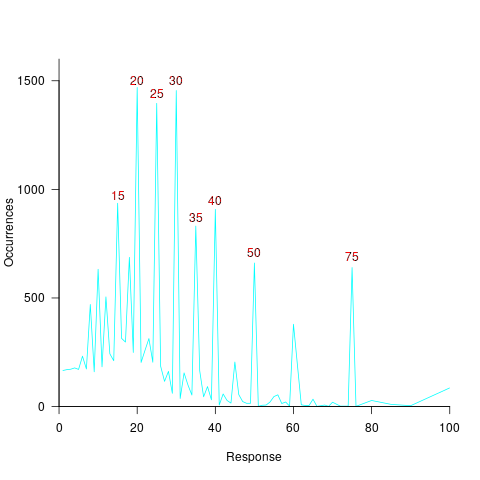The commonly occurring values that appear in software estimation data are structured as fractions of units of time, e.g., 0.5 hours, or 1 hour or 1 day (appearing in the data as 7 hours). The only structure available to experimental subjects was subdivisions of powers of 10 (i.e., 10 and 100).

Analysing the responses by subject shows that each subject had their own set of preferred round numbers.

To summarize: The results from an experiment investigating the interface between the two human number systems contains three patterns seen in software estimation data, i.e., power law relationship between actual and estimate, individual differences in over/underestimating, and extensive use of round numbers.

Izard’s second experiment limited response values to prespecified values (i.e., one to 10 and multiples of 10), and gave a calibration example after each block of 46 trials. The calibration example improved performance, and the use of round numbers as prespecified response values had the effect of removing spikes from the response counts (which were relatively smooth; code+data)).

We now have circumstantial evidence that software developers are using the Approximate Number System when making software estimates. We will have to wait for brain images from a developer in an MRI scanner, while estimating a software task, to obtain more concrete proof that the ANS is involved in the process. That is, are the areas of the brain thought to be involved in the ANS (e.g., the intraparietal sulcus) active during software estimation?

## Actual implementation times are often round numbers

To what extent do developers consciously influence the time taken to actually complete a task?

If the time estimated to complete a task is rather generous, a developer has the opportunity to follow Parkinson’s law (i.e., “work expands so as to fill the time available for its completion”), or if the time is slightly less than appears to be required, they might work harder to finish within the estimated time (like some marathon runners have a target time)?

The use of round numbers are a prominent pattern seen in task estimation times.

If round numbers appeared more often in the actual task completion time than would be expected by chance, it would suggest that developers are sometimes working to a target time. The following plot shows the number of tasks taking a given amount of actual time to complete, for project 615 in the CESAW dataset (similar patterns are present in the actual times of other projects; code+data):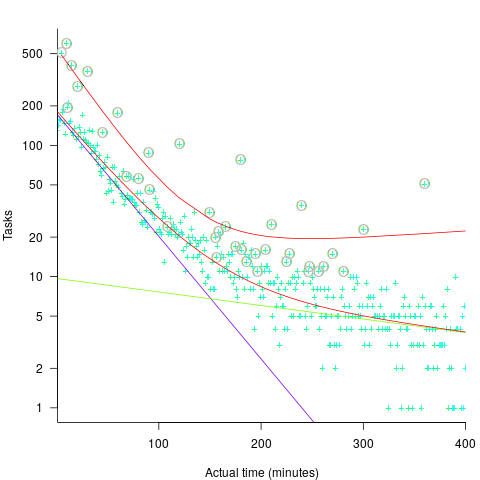The red lines are a fitted bi-exponential distribution to the ‘spike’ (i.e., round numbers, circled in grey) and non-spike points (spikes automatically selected, see code for details), green and purple lines are the two components of the non-spike fit.

Tasks are not always started and completed in one continuous work session, work may be spread over multiple work sessions; the CESAW data includes the start/end time of every work session associated with each task (85% of tasks involve more than one work session, for project 615). The following plots are based on work sessions, rather than tasks, for tasks worked on over two (left) and three (right) sessions; colored lines denote session ordering within a task (code+data):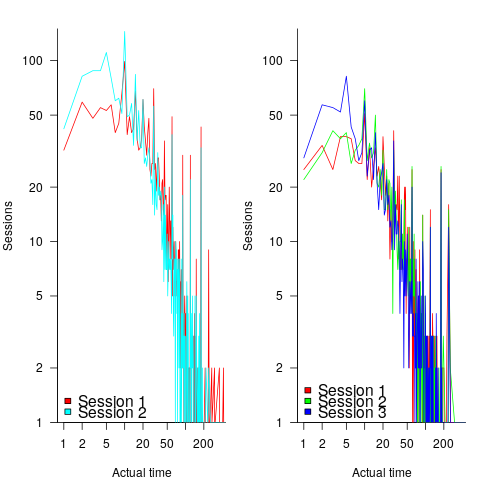Shorter sessions dominate for the last session of task implementation, and spikes in the counts indicate the use of round numbers in all session positions (e.g., 180 minutes, which may be half a day).

Perhaps round number work session times are a consequence of developers using round number wall-clock times to start and end work sessions. The plot below shows (left) the number of work sessions starting at a given number of minutes past the hour, and (right) the number of work sessions ending at a given number of minutes past the hour; both for project 615 (code+data):The arrow (green) shows the direction of the mean, and the almost invisible interior line shows that the length of the mean is almost zero. The five-minute points have slightly more session starts/ends than the surrounding minute values, but are more like bumps than spikes. The start of the hour, and 30-minutes, have prominent spikes, which might be caused by the start/end of the working day, and start/end of the lunch break.

Five-minutes is a convenient small rounding interval to either expand implementation time, or to target as a completion time. The following plot shows, for each of the 47 individuals working on project 615, the number of actual session times and the number exactly divisible by five. The green line shows the case where every actual is divisible by five, the purple line where 20% are divisible by five (expected for unbiased timing), the dashed purple lines show one standard deviation, the blue/green line is a fitted regression model (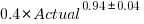) (code+data):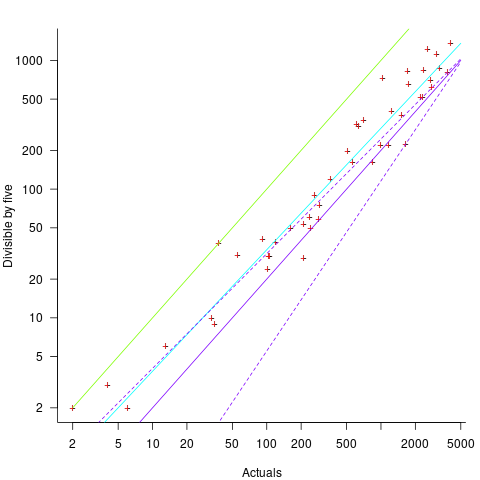It appears that on average, five-minute session times occur twice as often as expected by chance; two individuals round all their actual session times (ok, it’s not that unlikely for the person with just two sessions).

Does it matter that some developers have a preference for using round numbers when recording time worked?

The use of round numbers in the recording of actual work sessions will inflate the total actual time for most tasks (because most tasks involve more than one session, and assuming that most rounding is not caused by developers striving to meet a target). The amount of error introduced is probably a lot less than the time variability caused by other implementation factors (I have yet to do the calculation).

I see the use of round numbers as a means of unpicking developer work habits.

Given the difficulty of getting developers to record anything, requiring them to record to minute-level accuracy appears at best optimistic. Would you work for a manager that required this level of effort detail (I know there is existing practice in other kinds of jobs)?

## Actual implementation times are often round numbers

To what extent do developers consciously influence the time taken to actually complete a task?

If the time estimated to complete a task is rather generous, a developer has the opportunity to follow Parkinson’s law (i.e., “work expands so as to fill the time available for its completion”), or if the time is slightly less than appears to be required, they might work harder to finish within the estimated time (like some marathon runners have a target time)?

The use of round numbers are a prominent pattern seen in task estimation times.

If round numbers appeared more often in the actual task completion time than would be expected by chance, it would suggest that developers are sometimes working to a target time. The following plot shows the number of tasks taking a given amount of actual time to complete, for project 615 in the CESAW dataset (similar patterns are present in the actual times of other projects; code+data):The red lines are a fitted bi-exponential distribution to the ‘spike’ (i.e., round numbers, circled in grey) and non-spike points (spikes automatically selected, see code for details), green and purple lines are the two components of the non-spike fit.

Tasks are not always started and completed in one continuous work session, work may be spread over multiple work sessions; the CESAW data includes the start/end time of every work session associated with each task (85% of tasks involve more than one work session, for project 615). The following plots are based on work sessions, rather than tasks, for tasks worked on over two (left) and three (right) sessions; colored lines denote session ordering within a task (code+data):Shorter sessions dominate for the last session of task implementation, and spikes in the counts indicate the use of round numbers in all session positions (e.g., 180 minutes, which may be half a day).

Perhaps round number work session times are a consequence of developers using round number wall-clock times to start and end work sessions. The plot below shows (left) the number of work sessions starting at a given number of minutes past the hour, and (right) the number of work sessions ending at a given number of minutes past the hour; both for project 615 (code+data):The arrow (green) shows the direction of the mean, and the almost invisible interior line shows that the length of the mean is almost zero. The five-minute points have slightly more session starts/ends than the surrounding minute values, but are more like bumps than spikes. The start of the hour, and 30-minutes, have prominent spikes, which might be caused by the start/end of the working day, and start/end of the lunch break.

Five-minutes is a convenient small rounding interval to either expand implementation time, or to target as a completion time. The following plot shows, for each of the 47 individuals working on project 615, the number of actual session times and the number exactly divisible by five. The green line shows the case where every actual is divisible by five, the purple line where 20% are divisible by five (expected for unbiased timing), the dashed purple lines show one standard deviation, the blue/green line is a fitted regression model () (code+data):It appears that on average, five-minute session times occur twice as often as expected by chance; two individuals round all their actual session times (ok, it’s not that unlikely for the person with just two sessions).

Does it matter that some developers have a preference for using round numbers when recording time worked?

The use of round numbers in the recording of actual work sessions will inflate the total actual time for most tasks (because most tasks involve more than one session, and assuming that most rounding is not caused by developers striving to meet a target). The amount of error introduced is probably a lot less than the time variability caused by other implementation factors (I have yet to do the calculation).

I see the use of round numbers as a means of unpicking developer work habits.

Given the difficulty of getting developers to record anything, requiring them to record to minute-level accuracy appears at best optimistic. Would you work for a manager that required this level of effort detail (I know there is existing practice in other kinds of jobs)?

## Actual implementation times are often round numbers

To what extent do developers consciously influence the time taken to actually complete a task?

If the time estimated to complete a task is rather generous, a developer has the opportunity to follow Parkinson’s law (i.e., “work expands so as to fill the time available for its completion”), or if the time is slightly less than appears to be required, they might work harder to finish within the estimated time (like some marathon runners have a target time)?

The use of round numbers are a prominent pattern seen in task estimation times.

If round numbers appeared more often in the actual task completion time than would be expected by chance, it would suggest that developers are sometimes working to a target time. The following plot shows the number of tasks taking a given amount of actual time to complete, for project 615 in the CESAW dataset (similar patterns are present in the actual times of other projects; code+data):The red lines are a fitted bi-exponential distribution to the ‘spike’ (i.e., round numbers, circled in grey) and non-spike points (spikes automatically selected, see code for details), green and purple lines are the two components of the non-spike fit.

Tasks are not always started and completed in one continuous work session, work may be spread over multiple work sessions; the CESAW data includes the start/end time of every work session associated with each task (85% of tasks involve more than one work session, for project 615). The following plots are based on work sessions, rather than tasks, for tasks worked on over two (left) and three (right) sessions; colored lines denote session ordering within a task (code+data):Shorter sessions dominate for the last session of task implementation, and spikes in the counts indicate the use of round numbers in all session positions (e.g., 180 minutes, which may be half a day).

Perhaps round number work session times are a consequence of developers using round number wall-clock times to start and end work sessions. The plot below shows (left) the number of work sessions starting at a given number of minutes past the hour, and (right) the number of work sessions ending at a given number of minutes past the hour; both for project 615 (code+data):The arrow (green) shows the direction of the mean, and the almost invisible interior line shows that the length of the mean is almost zero. The five-minute points have slightly more session starts/ends than the surrounding minute values, but are more like bumps than spikes. The start of the hour, and 30-minutes, have prominent spikes, which might be caused by the start/end of the working day, and start/end of the lunch break.

Five-minutes is a convenient small rounding interval to either expand implementation time, or to target as a completion time. The following plot shows, for each of the 47 individuals working on project 615, the number of actual session times and the number exactly divisible by five. The green line shows the case where every actual is divisible by five, the purple line where 20% are divisible by five (expected for unbiased timing), the dashed purple lines show one standard deviation, the blue/green line is a fitted regression model () (code+data):It appears that on average, five-minute session times occur twice as often as expected by chance; two individuals round all their actual session times (ok, it’s not that unlikely for the person with just two sessions).

Does it matter that some developers have a preference for using round numbers when recording time worked?

The use of round numbers in the recording of actual work sessions will inflate the total actual time for most tasks (because most tasks involve more than one session, and assuming that most rounding is not caused by developers striving to meet a target). The amount of error introduced is probably a lot less than the time variability caused by other implementation factors (I have yet to do the calculation).

I see the use of round numbers as a means of unpicking developer work habits.

Given the difficulty of getting developers to record anything, requiring them to record to minute-level accuracy appears at best optimistic. Would you work for a manager that required this level of effort detail (I know there is existing practice in other kinds of jobs)?

## Estimating using a granular sequence of values

When asked for an estimate of the time needed to complete a task, should developers be free to choose any numeric value, or should they be restricted to selecting from a predefined set of values (e.g, the Fibonacci numbers, or T-shirt sizes)?

Allowing any value to be chosen would appear to provide the greatest flexibility to make an accurate estimate. However, estimating is an intrinsically uncertain process (i.e., the future is unknown), and it is done by people with varying degrees of experience (which might be used to help guide their prediction about the future).

Restricting the selection process to one of the values in a granular sequence of numbers has several benefits, including:

• being able to adjust the gaps between permitted values to match the likely level of uncertainty in the task effort, or the best accuracy resolution believed possible,
• reducing the psychological stress of making an estimate, by explicitly giving permission to ignore the smaller issues (because they are believed to require a total effort that is less than the sequence granularity),
• helping to maintain developer self-esteem, by providing a justification when an estimate turning out to be inaccurate, e.g., the granularity prevented a more accurate estimate being made.

Is there an optimal sequence of granular values to use when making task estimates for a project?

The answer to this question depends on what is attempting to be optimized.

Given how hard it is to get people to produce estimates, the first criterion for an optimal sequence has to be that people are willing to use it.

I have always been struck by the ritualistic way in which the Fibonacci sequence is described by those who use it to make estimates. Rituals are an effective technique used by groups to help maintain members’ adherence to group norms (one of which might be producing estimates).

A possible reason for the tendency to use round numbers might estimate-values is that this usage is common in other social interactions involving numeric values, e.g., when replying to a request for the time of day.

The use of round numbers, when developers have the option of selecting from a continuous range of values, is a developer imposed granular sequence. What form do these round number sequences take?

The plot below shows the values of each of the six most common round number estimates present in the BrightSquid, SiP, and CESAW (project 615) effort estimation data sets, plus the first six Fibonacci numbers (code+data):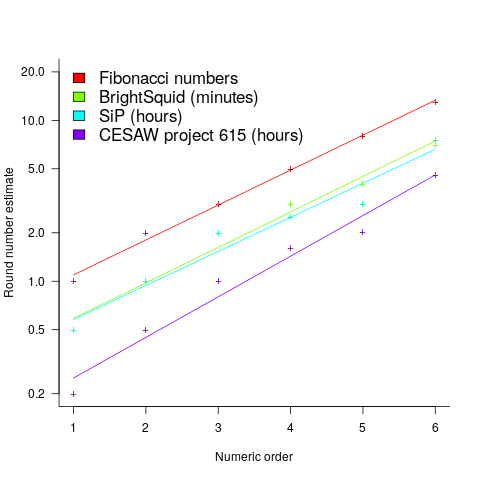The lines are fitted regression models having the form: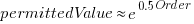(there is a small variation in the value of the constant; the smallest value for project 615 was probably calculated rather than being human selected).

This plot shows a consistent pattern of use across multiple projects (I know of several projects that use Fibonacci numbers, but don’t have any publicly available data). Nothing is said about this pattern being (near) optimal in any sense.

The time unit of estimation for this data was minutes or hours. Would the equation have the same form if the time unit was days, would the constant still be around. I await the data needed to answer this question.

This brief analysis looked at granular sequences from the perspective of the distribution of estimates made. Perhaps it makes more sense to base a granular estimation sequence on the distribution of actual task effort. A topic for another post.

## Estimating using a granular sequence of values

When asked for an estimate of the time needed to complete a task, should developers be free to choose any numeric value, or should they be restricted to selecting from a predefined set of values (e.g, the Fibonacci numbers, or T-shirt sizes)?

Allowing any value to be chosen would appear to provide the greatest flexibility to make an accurate estimate. However, estimating is an intrinsically uncertain process (i.e., the future is unknown), and it is done by people with varying degrees of experience (which might be used to help guide their prediction about the future).

Restricting the selection process to one of the values in a granular sequence of numbers has several benefits, including:

• being able to adjust the gaps between permitted values to match the likely level of uncertainty in the task effort, or the best accuracy resolution believed possible,
• reducing the psychological stress of making an estimate, by explicitly giving permission to ignore the smaller issues (because they are believed to require a total effort that is less than the sequence granularity),
• helping to maintain developer self-esteem, by providing a justification when an estimate turning out to be inaccurate, e.g., the granularity prevented a more accurate estimate being made.

Is there an optimal sequence of granular values to use when making task estimates for a project?

The answer to this question depends on what is attempting to be optimized.

Given how hard it is to get people to produce estimates, the first criterion for an optimal sequence has to be that people are willing to use it.

I have always been struck by the ritualistic way in which the Fibonacci sequence is described by those who use it to make estimates. Rituals are an effective technique used by groups to help maintain members’ adherence to group norms (one of which might be producing estimates).

A possible reason for the tendency to use round numbers might estimate-values is that this usage is common in other social interactions involving numeric values, e.g., when replying to a request for the time of day.

The use of round numbers, when developers have the option of selecting from a continuous range of values, is a developer imposed granular sequence. What form do these round number sequences take?

The plot below shows the values of each of the six most common round number estimates present in the BrightSquid, SiP, and CESAW (project 615) effort estimation data sets, plus the first six Fibonacci numbers (code+data):The lines are fitted regression models having the form:(there is a small variation in the value of the constant; the smallest value for project 615 was probably calculated rather than being human selected).

This plot shows a consistent pattern of use across multiple projects (I know of several projects that use Fibonacci numbers, but don’t have any publicly available data). Nothing is said about this pattern being (near) optimal in any sense.

The time unit of estimation for this data was minutes or hours. Would the equation have the same form if the time unit was days, would the constant still be around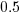. I await the data needed to answer this question.

This brief analysis looked at granular sequences from the perspective of the distribution of estimates made. Perhaps it makes more sense to base a granular estimation sequence on the distribution of actual task effort. A topic for another post.

## Estimating in round numbers

People tend to use round numbers. When asked the time, the response is often rounded to the nearest 5-minute or 15-minute value, even when using a digital watch; the speaker is using what they consider to be a relevant level of accuracy.

When estimating how long it will take to perform a task, developers tend to use round numbers (based on three datasets). Giving what appears to be an overly precise value could be taken as communicating extra information, e.g., an estimate of 1-hr 3-minutes communicates a high degree of certainty (or incompetence, or making a joke). If the consumer of the estimate is working in round numbers, it makes sense to give a round number estimate.

Three large software related effort estimation datasets are now available: the SiP data contains estimates made by many people, the Renzo Pomodoro data is one person’s estimates, and now we have the Brightsquid data (via the paper “Utilizing product usage data for requirements evaluation” by Hemmati, Didar Al Alam and Carlson; I cannot find an online pdf at the moment).

The plot below shows the total number of tasks (out of the 1,945 tasks in the Brightsquid data) for which a given estimate value was recorded; peak values shown in red (code+data):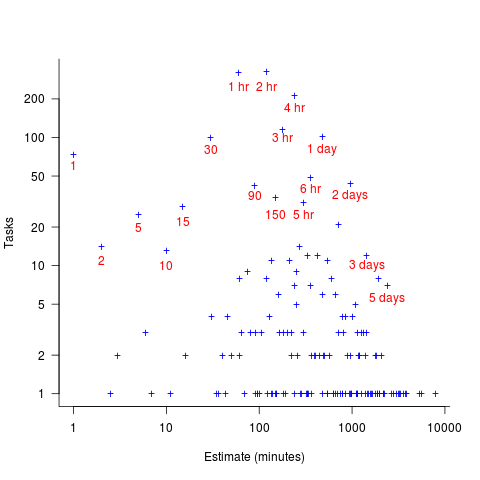Why are there estimates for tasks taking less than 30 minutes? What are those 1 minute tasks (are they typos, where the second digit was omitted and the person involved simply create a new estimate without deleting the original)? How many of those estimate values appearing once are really typos, e.g., 39 instead of 30? Does the task logging system used require an estimate before anything can be done? Unfortunately I don’t have access to the people involved. It does look like this data needs some cleaning.

There are relatively few 7-hour estimates, but lots for 8-hours. I’m assuming the company works an 8-hour day (the peak at 4-hours, rather than three, adds weight to this assumption).